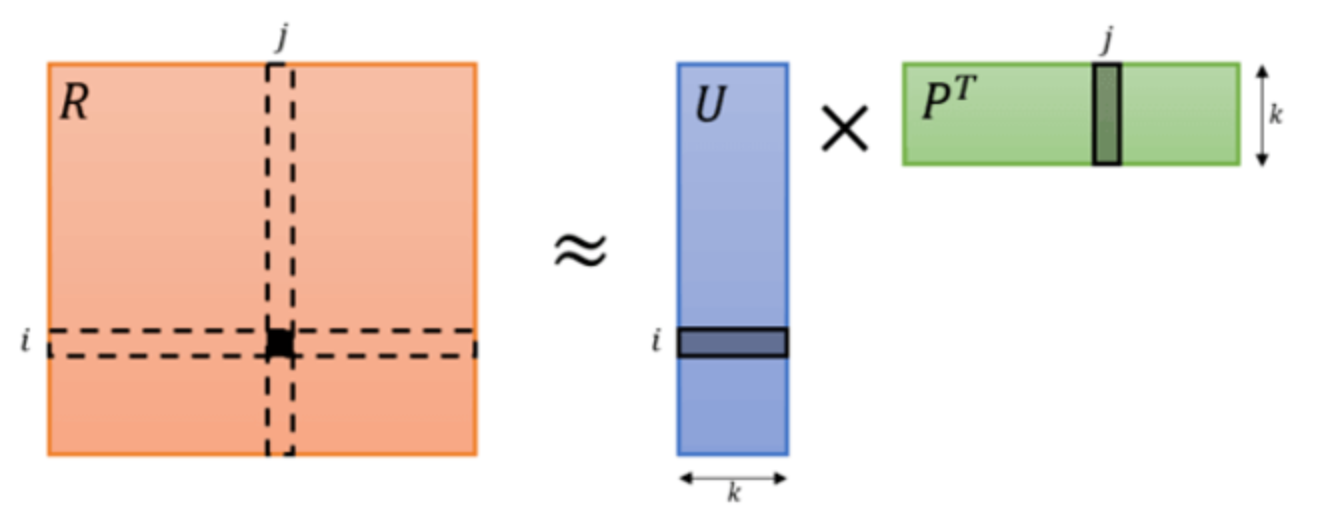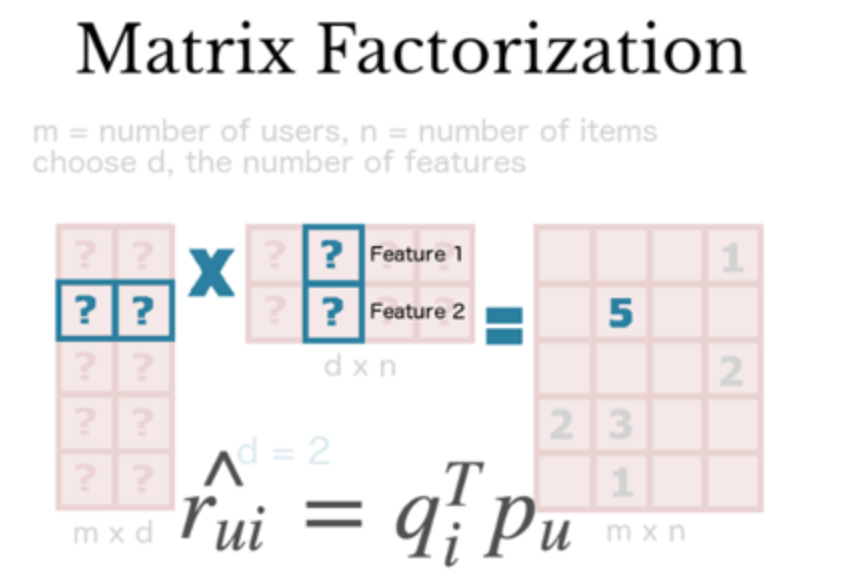### 一、矩阵分解### 二、矩阵分解的优缺点

• 泛化能力强。在一定程度上解决了数据稀疏的问题。
• 空间复杂度低。不需再存储协同过滤模型所需的“庞大“用户相似性或物品相似性矩阵，只需存储用户和物品隐向量空间复杂度由 $n^2$ 级别降低到 $(n+m)*k$ 级别。
• 更好的扩展性和灵活性。矩阵分解最终产出的是用户和物品隐向量，这其实与深度学习中的Embedding思想不谋而合，因此矩阵分解的结果也非常便于与其他特征进行组合和拼接，与深度学习网络进行无缝组合。

• 矩阵分解不方便加入用户、物品、上下文相关的特征，丧失了利用很多有效信息的机会。
• 在缺乏用户历史行为时，无法进行有效的推荐。
• 模型训练比较费时。
• 推荐结果不具有很好的可解释性，分解出来的用户和物品矩阵的每个维度无法用现实生活中的概念来解释，只能理解为潜在语义空间。

### 三、矩阵分解相关算法

#### 3.1 奇异值分解SVD

• SVD 要求矩阵是稠密的，而现实场景中的共现矩阵是稀疏的，有大量空白，无法直接使用 SVD 分解。要想使用 SVD，必须对评分矩阵中的缺失值进行简单的补全，比如用全局平均值或者用用户物品平均值补全，得到补全后的矩阵。接着可以用 SVD 分解并降维。但填充本身会造成很多问题，其一，填充大大增加了数据量，增加了算法复杂度。其二，简单粗暴的数据填充很容易造成数据失真。
• 传统奇异值分解的计算复杂度达到了 $O(mn^2)$ 的级别，这对于商品数量动辄上百万、用户数量往往上千万的互联网场景来说几乎是不可接受的。

#### 3.2 Funk-SVD

$Simon Funk$ 在博客上公开发表了一个只考虑已有评分记录的矩阵分解方法，称为 $Funk-SVD$，也就是被 $Yehuda Koren$ 称为隐语义模型的矩阵分解方法。它的出发点为，既然将一个矩阵做 SVD 分解成 3 个矩阵很耗时，同时还面临稀疏的问题，那么我们能不能避开稀疏问题，同时只分解成两个矩阵呢？也就是说，现在期望我们的矩阵$M$ 这样进行分解：

$$M_{m×n}=P_{m×k}^TQ_{k×n}$$

$$\sum_{i,j}(m_{ij}-q_j^Tp_i)^2$$

$$arg\mathop{min}\limits_{p_i,q_j}\sum_{(i,j)\in K}(m_{ij}-q_j^Tp_i)^2+\lambda(||p_i||^2_2+||q_j||^2_2)$$

$$\frac{∂J}{∂p_i} = -2(m_{ij}-q_j^Tp_i)q_j+2\lambda p_i$$

$$\frac{∂J}{∂q_j} = -2(m_{ij}-q_j^Tp_i)p_i+2\lambda q_j$$

$$p_i = p_i + \alpha ((m_{ij}-q_j^Tp_i)q_j-\lambda p_i)$$

$$q_j = q_j + \alpha ((m_{ij}-q_j^Tp_i)p_i-\lambda q_j)$$

#### 3.3 Bias-SVD

$$r_{ui} = \mu + b_i + b_u + q_j^Tp_i$$

• 训练集中所有评分记录的全局平均数 $\mu$ ，表示了训练数据的总体评分情况，对于固定的数据集，它是一个常数。
• 用户偏置 $b_u$，独立于物品特征的因素，表示某一特定用户的打分习惯。例如，对于批判性用户对于自己的评分比较苛刻，倾向于打低分；而乐观型用户则打分比较保守，总体打分要偏高。
• 物品偏置 $b_i$ ，特立于用户兴趣的因素，表示某一特定物品得到的打分情况。以电影为例，好片获得的总体评分偏高，而烂片获得的评分普遍偏低，物品偏置捕获的就是这样的特征。

$$arg\mathop{min}\limits_{p_i,q_j}\sum_{(i,j)\in K}(m_{ij}-q_j^Tp_i-\mu-b_i-b_u)^2+\lambda(||p_i||^2_2+||q_j||^2_2+||b_i||^2_2+||b_u||^2_2)$$

### 四、基于Pytorch的矩阵分解微信支付宝